# Introduction to Statistics for Research: Inference about means

## Comparing the means of two independent large samples

In healthcare research, we more often want to compare groups of subjects than use a single sample to estimate the mean in the population. For example, Christensen et al. (2004) compared interventions for depression delivered using the internet. They recruited 525 people with symptoms of depression identified in a survey. These were randomly allocated to a website, BluePages, offering information about depression (n = 166) or a cognitive behaviour therapy website, MoodGYM, (n = 182), or a control intervention using an attention placebo (n = 178). The main outcome measure was the Center for Epidemiologic Studies depression scale. This consists of 20 questions scored 0 (not depressed) to 3 (depressed) and summed, giving a score between 0 and 60. The means and standard deviations of the falls in depression score for MoodGYM and for Controls are shown in Table 1.

Table 1. Mean fall in depression score after six weeks by treatment group for patients with depression (Christensen et al., 2004)
Treatment Number Fall in scores
Mean SD
MoodGYM 182 4.2 9.1
Controls 178 1.0 8.4

We can find a confidence interval for difference between the means of two independent samples. For example, we shall compare the mean fall in score for MoodGYM with Control. The difference between the means, MoodGYM minus Control, = 4.2 – 1.0 = 3.2. We can find the standard error for the difference by squaring the standard error of each mean, adding, and taking the square root. This only works when the groups are independent. If we were to do it for paired data like the before and after measurements above, the standard error might be much too large. For BluePages and MoodGYM, we have

√(0.672 + 0.372) = 0.92.

The 95% CI is then given by 3.2 ” 1.96 × 0.92 to 3.2 + 1.96 × 0.92 = 1.40 to 5.00.

We can also do a test of the null hypothesis that in the population the difference between the means is zero against the alternative hypothesis that the difference in the population is not zero. As for the paired example above, because we have a large sample the observed difference minus the population difference then divided by the estimated standard error of the difference should be an observation from a Standard Normal distribution. If the null hypothesis were true, the population difference would be zero. The test statistic is observed difference divided by its standard error, z = 3.2/0.92 = 3.48. The probability of an observation from the Standard Normal distribution being as far from its expected value, zero, as 3.48 is P=0.0005. Hence the difference is highly significant.

We can tell this from the 95% confidence interval, also, as this does not include zero, the null hypothesis value for the difference. This is the large sample Normal distribution test or z test for the means of two independent groups.

The large sample Normal method for comparing two means requires two assumptions about the data.

• The observations and groups are independent. We should not have links between observations in the two groups, such as a matched study where each subject in one group is matched, e.g. by age and sex, with a subject in the other group.
• The samples are large enough for the standard errors to be well estimated and for the means to be observations from Normal distributions. My rule of thumb is that for a single sample there should be at least 100 observations and for two samples at least 50 in each.

Some computer programs do not do large sample z tests directly. You have to use the command for a one sample or paired t test, or for a two-sample t test with unequal variances. I describe these below. For large samples, they give the same answers as the z tests.

## The two sample t method

This is also called the unpaired t method or unpaired t test, the two group t method, or Student's two sample t test. It enables us to estimate the difference between means or test the null hypothesis of no difference in the population, even when the samples are small.

Our example is a comparison of blood glucose (mmol/L) measured in a two groups of small neonates given different infant feed formulae (Table 2).

 Formula 1 Formula 2 Number 1.2   3.5   3.9   4.2   4.8     2.8   3.7   3.9   4.3   5.7   3.1   3.8   4.0   4.4   5.9   3.1   3.9   4.0   4.4     3.2   3.9   4.2   4.7 2.8   3.3   4.0   4.6     2.9   3.4   4.0   4.7   3.1   3.6   4.2   5.0   3.1   3.7   4.3   5.1   3.1   3.9   4.5   6.8 23 20 3.94 4.01 0.95 0.96

The data are shown graphically in Figure 1.

Figure 1. Scatter diagram showing blood glucose in two groups of small neonates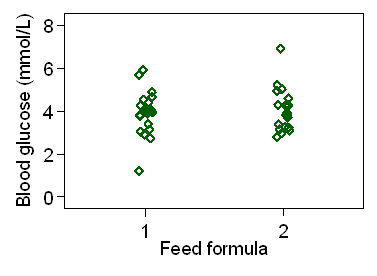d

The samples are small, only 23 on formula 1 and 20 on Formula 2, so we cannot use the large sample Normal method. The standard error will not be sufficiently well estimated.

For the two-sample t method, we must make three assumptions about the data:

• The observations and groups are independent.
• The observations come from Normal distributions,
• The distributions in the two populations have the same variance. (N.B. The populations, not the samples from them, have the same variance.)

If the distributions in the two populations have the same variance, we need only one estimate of variance. We call this the common or pooled variance estimate. It is a weighted average of the two sample variances, weighted by the degrees of freedom. The degrees of freedom for this common variance estimate are the number of observations minus 2. We then use this common estimate of variance to estimate the standard error of the difference between the means.

For the infant formula example, the common variance of blood glucose = 0.91135, SD = 0.95 mmol/L, d.f. = 23 + 20  2 = 41. The difference (Formula 1 – Formula 2) = 4.01 – 3.94 = 0.07 mmol/L. The standard error of the difference, calculated from the pooled variance and the numbers in the two groups, is 0.29 mmol/L.

Then the 95% confidence interval for difference is given by 0.07 – ? × 0.29 to 0.07 + ? × 0.29.

For a large sample confidence interval, the number indicated by "?" would come from the Standard Normal distribution and would be 1.96. Here it comes not from the Normal distribution but a different distribution called the t distribution.

When samples are small, we cannot apply the large sample Normal distribution methods safely. This problem was tackled by a statistician who published under the pseudonym Student, because his employers would not allow him to publish the results of his work. The probability distribution which he discovered is known as Student's t distribution as a result and the methods which use it as Student's t tests.

We have seen that when the sample is large, the observed sample mean minus the population mean divided by the standard error follows the Standard Normal distribution. When the sample is small this is not so. The distribution followed depends on the distribution of the observations themselves, unlike the large sample case where this is irrelevant. We have to assume that the data themselves come from a population which follows a Normal distribution. We have seen that some naturally occurring variables do this and some do not. We shall see in the next lecture that many variables which do not follow a Normal distribution can be made to do so by changing the way in which we look at them, using a transformation such as the logarithm. When the observations come from a population which follows a Normal distribution, then the sample mean minus the population mean divided by the standard error of the mean follows Student's t distribution, or simply the t distribution. Student's t distribution may be defined as the distribution which this ratio would follow.

Like the Normal distribution, Student's t distribution is a family of distributions rather than just one. This family has only has one parameter, the number which tells us with which member of the family of t distributions we are dealing. This is called the degrees of freedom. We have already used this term in the calculation of variances and standard deviations. The degrees of freedom of the t distribution is equal to the degrees of freedom of the standard deviation used in the calculation of the standard error.

Figure 2 shows some members of the Student's t distribution family.

Figure 2. Student's t distribution with 1, 4, and 20 degrees of freedom, with the Standard Normal distribution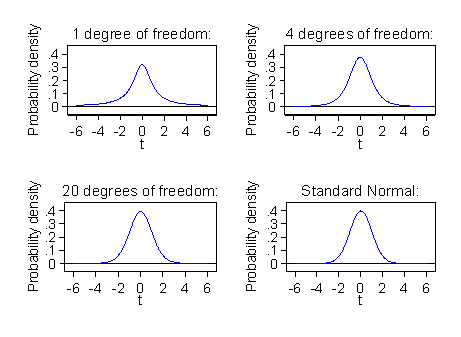d

When the degrees of freedom are small, corresponding to small samples, the t distribution has much longer tails than the Normal. This reflects the greater uncertainty in the standard error of the mean. As the degrees of freedom and hence the related sample size gets bigger, the t distribution gets closer and closer to the Standard Normal distribution. The t distribution reaches the Normal distribution in theory when the sample is infinitely large. In practice, it is difficult to tell the Normal and t distributions apart at about 30 degrees of freedom.

Like the Normal, the t distribution has no simple formulae for its probabilities. Instead we used numerical approximations to calculate the number which replaces 1.96 in confidence interval calculations and the P values in significance tests. If we do these calculations using one of the many computer programs available, the program will calculate these for us. For the purposes of illustration, I shall also give a short table of the distribution for different degrees of freedom (Table 3).

Table 3. Two tailed probability points of the t Distribution
D.f. Probability         D.f. Probability
0.10 0.05 0.01 0.001 0.10 0.05 0.01 0.001
(10%) (5%) (1%) (0.1%) (10%) (5%) (1%) (0.1%)
1 6.31 12.70 63.66 636.62 16 1.75 2.12 2.92 4.02
2 2.92 4.30 9.93 31.60 17 1.74 2.11 2.90 3.97
3 2.35 3.18 5.84 12.92 18 1.73 2.10 2.88 3.92
4 2.13 2.78 4.60 8.61 19 1.73 2.09 2.86 3.88
5 2.02 2.57 4.03 6.87 20 1.73 2.09 2.85 3.85
6 1.94 2.45 3.71 5.96 21 1.72 2.08 2.83 3.82
7 1.90 2.36 3.50 5.41 22 1.72 2.07 2.82 3.79
8 1.86 2.31 3.36 5.04 23 1.71 2.07 2.81 3.77
9 1.83 2.26 3.25 4.78 24 1.71 2.06 2.80 3.75
10 1.81 2.23 3.17 4.59 25 1.71 2.06 2.79 3.73
11 1.80 2.20 3.11 4.44 30 1.70 2.04 2.75 3.65
12 1.78 2.18 3.06 4.32 40 1.68 2.02 2.70 3.55
13 1.77 2.16 3.01 4.22 60 1.67 2.00 2.66 3.46
14 1.76 2.15 2.98 4.14 120 1.66 1.98 2.62 3.37
15 1.75 2.13 2.95 4.07 1.65 1.96 2.58 3.29
D.f. = Degrees of freedom
∞ = infinity, t is the same as the Standard Normal Distribution

For each of the degrees of freedom given, Table 2 gives the value which will be exceeded, in either positive or negative direction, with the given probability. For example, Figure 3 shows the 5% two sided probability points of the t distribution with 4 degrees of freedom.

Figure 3. 5% probability points of the t distribution with 4 degrees of freedom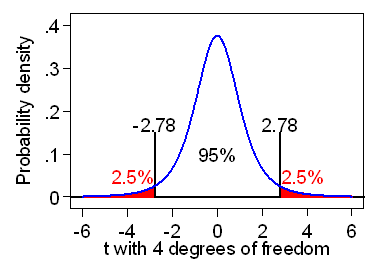d

We can use Student's t distribution to replace the Normal distribution in confidence interval and significance tests for small samples. To do this we must be able to assume that the observations themselves come from a Normal distribution, plus other assumptions for different applications as described below.

Then the 95% confidence interval for difference is given by 0.07 – t × 0.29 to 0.07 + t × 0.29. t comes from the t distribution with 41 degrees of freedom. It is the 5% point of the distribution, because 5% of observations will be further from zero than t, 95% will be closer to zero than t. For 41 degrees of freedom, t = 2.02. Hence the 95% CI is 0.07 –2.02 × 0.29 to 0.07 + 2.02 × 0.29 = –0.52 to +0.66 mmol/L.

We can also carry out a test of significance, testing the null hypothesis that in the population the difference between means = 0. We take the observed difference divided by its standard error and, if the null hypothesis were true, this would be an observation from the t distribution with 41 degrees of freedom. We have difference/SE = 0.07/0.95 = 0.24.

From Table 2, the probability of such an extreme value is greater than 0.10. If we use a good computer program, this will calculate the P value for us more accurately. In this case we get P = 0.8228, which we report as P = 0.8.

We can check the assumption that energy expenditure follows a Normal distribution in each population by histograms and Normal plots. Figure 4 shows histograms for each group.

Figure 4. Histograms of blood glucose in two groups of neonates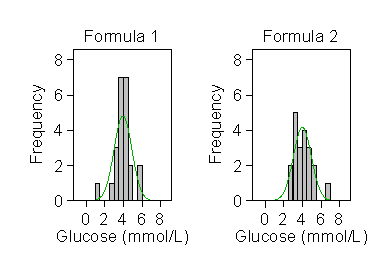d

There are not enough observations to judge whether the data follow Normal distributions. We can improve matters by combining the two groups. The distribution would be affected by any difference between the means, perhaps even becoming bimodal. We get round this by subtracting the group mean from each observation to give residuals. The residuals have mean = 0 in each group. We can then put them together to form a single distribution, as shown in Figure 5.

Figure 5. Distribution of residual blood glucose, with corresponding Normal distribution curve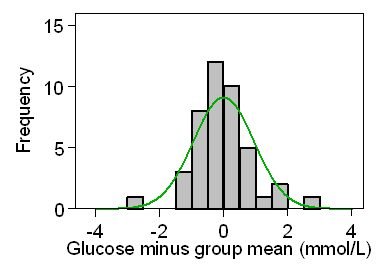d

This looks fairly symmetrical, but there are still only a few observations. We cannot really say whether the Normal distribution and the data have the same shape. There is a better graphical method to examine the fit of a Normal distribution to a set of data, the Normal quantile plot or Normal plot for short. A Normal plot is a plot of the observed data against the values which we would expect if the data actually followed a Normal distribution. Table 3 shows the results of the calculation for the blood glucose data for the Formula 2 group.

Table 3. Estimation of a Normal plot for blood glucose in the formula 2 group
Glucose
mmol/L
Standard Normal,
mean = 0, SD = 1
Normal with
mean = 4.01, SD = 0.96
2.8 –1.67 2.41
2.9 –1.31 2.75
3.1 –1.07 2.98
3.1 –0.88 3.16
3.1 –0.71 3.32
3.3 –0.57 3.46
3.4 –0.43 3.59
3.6 –0.30 3.71
3.7 –0.18 3.83
3.9 –0.06 3.95
4.0 0.06 4.06
4,0 0.18 4.18
4.2 0.30 4.30
4.3 0.43 4.42
4.5 0.57 4.55
4.6 0.71 4.69
4.7 0.88 4.85
5.0 1.07 5.03
5.1 1.31 5.26
6.8 1.67 5.60

First we put our observations into ascending order. There are 20 of them, and we ask what would be the expected values of the smallest observation from a sample of nine from a Normal distribution. For the Standard Normal distribution this is –1.67. (As usual, we skip the formulae because the computer program will do all this for us.) We expect the next up to be –1.31, the next to be –1.07, etc. The middle value is expected to be zero, the mean and median of the Standard Normal distribution. As we have an even number of observations, 20, we don't actually have a point at the median for this sample. We now convert these to a Normal distribution with the same mean and variance as the data by multiplying the Standard Normal value by the sample standard deviation and adding the sample mean. Thus we would expect the smallest of nine observations from a Normal distribution with mean = 4.01 and standard deviation = 0.96 to be –1.67 × 0.96 + 4.01 = 2.41. Compare this to the observed smallest value, which is 2.8. Inspecting Table 3 will show you that most of the observed glucose measurements and the glucose measurements we would expect if we had a Normal distribution are quite close.

We can now plot the observed glucose measurements against the glucose measurements which would be expected if data followed a Normal distribution. If the observed and expected are similar, observations should lie close to the line of equality, which joins points where the observed and expected would be equal, which we also draw on the graph. Figure 6 shows the Normal plot for the glucose measurements.

Figure 6. Normal plot for the glucose data, Formula 2 group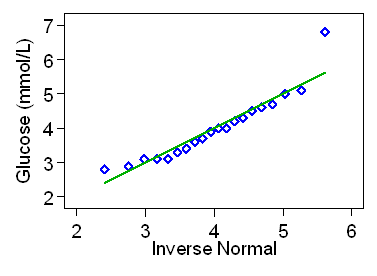d

Most of the observations are indeed close to the line, suggesting that the observations are quite close to a what we would expect from a Normal distribution.

If the points form a curve becoming steeper as glucose increases, this indicates positive skewness, If the points form a curve becoming less steep as glucose increases, this indicates negative skewness. Figure 6 shows a fairly good fit to the line apart from one outlying point. However, at the low end of glucose points are just above line, then just below it for most of its length, with the outlier above it. The curve does get steeper, indicating a bit of skewness in the data.

Figure 7 shows the Normal plot for residual blood glucose.

Figure 7. Normal plot for residual blood glucose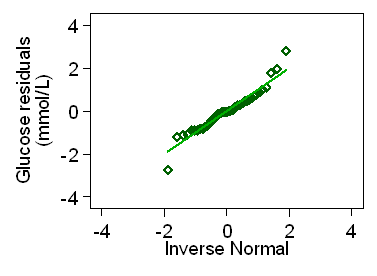d

The Normal plot conforms fairly well to the straight line, apart from a couple of slight outliers, confirming that the distribution is approximately Normal.

The other assumption is that the variances are the same in each population. For the blood glucose, Table 2 shows that the standard deviations are very similar in the two samples, being 0.95 mmol/L for the Formula 1 group and 0.96 mmol/L for the Formula 2 group. Figure 4 also shows a similar spread in the two groups.

We can also test the equality of variances, either with an F test or Levene's test. However, tests have the unfortunately property that they miss large differences for small samples, when differences might matter, and find them for large samples, when they matter much less. It is usually preferable to judge whether the assumption of uniform variance is plausible from the scatter plot (Figure 4).

Methods using the t distribution depend on some strong assumptions about the distributions from which the data come. In general, for two equal sized samples the t method is very resistant to deviations from Normality, though as the samples become less equal in size the approximation becomes less good. The most likely effect of skewness is that we lose power. P values are then too large and confidence intervals too wide. We can usually correct skewness by a transformation, as described in Week 5.

If we cannot assume uniform variance, the effect is usually small if the two populations are from a Normal Distribution. However, unequal variance is often associated with skewness in the data. When distributions are positively skew, the variability usually increases with increasing mean. This is the case for the energy expenditure, of course. In this case a transformation designed to correct one fault often tends to correct the other as well.

If distributions are Normal, we can use the Satterthwaite correction to the degrees of freedom, often called the two sample t method for unequal or unpooled variance.

If variances are unequal, we cannot estimate a common variance. Instead we use the large sample form of the standard error of the difference between means. We replace the t value for confidence intervals and significance tests by t with fewer degrees of freedom. The Satterthwaite degrees of freedom depend on the relative sizes of the variances. The larger variance dominates and if one is much larger than the other the degrees of freedom for that group are the only degrees of freedom.

For the blood glucose example, the standard error of the difference between means is 0.2923, the degrees of freedom = 41 (= 23 + 20  2). The unpooled standard error, found as for the comparison of two large sample means, is 0.2924, Satterthwaite's degrees of freedom = 40.1. This is almost unchanged because the variances here are almost the same. We round this down to 40 to use the t table. For this example, the t test for equal variances gives P = 0.8228, unequal variances also gives P = 0.8229.

The two sample t method is very robust to small departures from its assumptions, especially when the groups are of similar size, as here.

N.B. Satterthwaite's method is an approximation for use in unusual circumstances. The equal variance method is the standard t test.

## The paired t method

The paired t method is used when we have paired observations, such as the same subject before and after an intervention, the same subject receiving two different interventions as in a cross-over trial, or matched cases and controls in a case-control study. et al., 2004), In this trial, patients with chronic non-healing wounds were randomised to receive topical placental extract or to control. This was a before-and-after treatment study of the use of topical placental extract in 9 patients with non-healing wounds. Biopsies were assessed using the microscopic angiogenesis grading system (MAGS) score, which provides an index of how well small blood vessels are developing and hence of epithelial regeneration. High scores are good. The data in Table 4 show the MAGS score before and after treatment in a group 9 of the patients in the active treatment group.

Table 4. MAGS score before and after treatment with topical placental extract in 9 patients with non-healing wounds (Shukla et al., 2004)
MAGS score
before
MAGS score
after
Difference,
MAGS before
minus MAGS after
Average of
MAGS before
and MAGS after
20 32 12 26.0
31 47 16 39.0
34 43 9 38.5
39 43 4 41.0
43 55 12 49.0
45 52 7 48.5
49 61 12 55.0
51 55 4 53.0
63 71 8 67.0

We want to know whether we have evidence that mean MAGS score changed and what the average score might be. I have calculated the difference between the MAGS score after treatment and the MAGS score before treatment, i.e. the increase in the MAGS score.

The authors of the paper did not do any further analysis of these data, as they were all positive differences and the MAGS score clearly increases following treatment. We shall use them to estimate the mean increase in MAGS score. The mean and standard deviation of the increase in MAGS score are 9.33 and 4.03 respectively. We have 9 observations so the number of degrees of freedom for the calculation of the standard deviation is 9 - 1 = 8.

The standard error of the mean difference is 1.34. To estimate the 95% confidence interval for the mean from this small sample, we use the 5% point of the t distribution with 8 degrees of freedom. From the 8 degrees of freedom row in Table 2 this is 2.31. The 95% confidence interval is therefore the mean minus or plus 2.31 standard errors, 9.33 - 2.31 × 1.34 to 9.33 + 2.31 × 1.34, which gives us 6.2 to 12.4.

We can also test the null hypothesis that in the population the mean increase is zero. The test statistic is the mean divided by its standard error. This is 9.33/1.34 = 6.96. If we look in the 8 degrees of freedom row in Table 2, we see that this is larger than the largest number there, 5.04, which corresponds to a probability of 0.001. Hence we could say P<0.001. In practice, we would do this using a computer program, which gives us P = 0.0001. The difference is highly significant.

There are several assumptions which we must make about the data for the paired t method test to be valid:

• the observations must be independent, apart from the pairing,
• the differences must follow a Normal distribution,
• the mean and standard deviation of the differences must be constant, i.e. not related to the size of the measurement.

The first of these, independence, depends on the design. It is met for the MAGS data, because the pairs of data come from nine different subjects. The second can be tested by a Normal plot, as shown in Figure 8.

Figure 8. Normal plot for the increases in MAGS score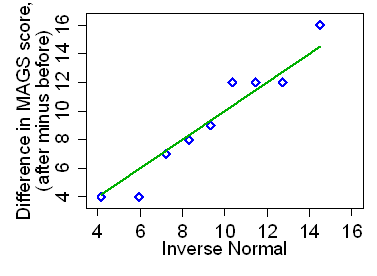d

This appears to fit the straight line quite well and there is no reason to suppose that the differences do not follow a Normal distribution. The third, that the mean and the variability are not related to the magnitude, can also be investigated graphically. We do a scatter plot of the difference against the average of the two observations, as in Figure 9.

Figure 9. Difference versus mean plot for the increases in MAGS scored

We do this because the average of the two measurements is the best estimate we have of the subject's true MAGS score over the period. Using only one of the measurements, either before or after, on the horizontal axis tends to produce spurious relationships between difference and magnitude. For the MAGS data, Figure 9 shows little evidence that either the mean difference or the variability of the differences is related to the magnitude of MAGS score for the subject.

## References

Christensen H, Griffiths KM, Jorm AF. (2004) Delivering interventions for depression by using the internet: randomised controlled trial. British Medical Journal 328, 265-268.

Shukla VK, Rasheed MA, Kumar M, Gupta SK, Pandey SS. (2004) A trial to determine the role of placental extract in the treatment of chronic non-healing wounds. Journal of Wound Care 13, 177-9.